• 《概率论基础》，复旦大学，李贤平，第三版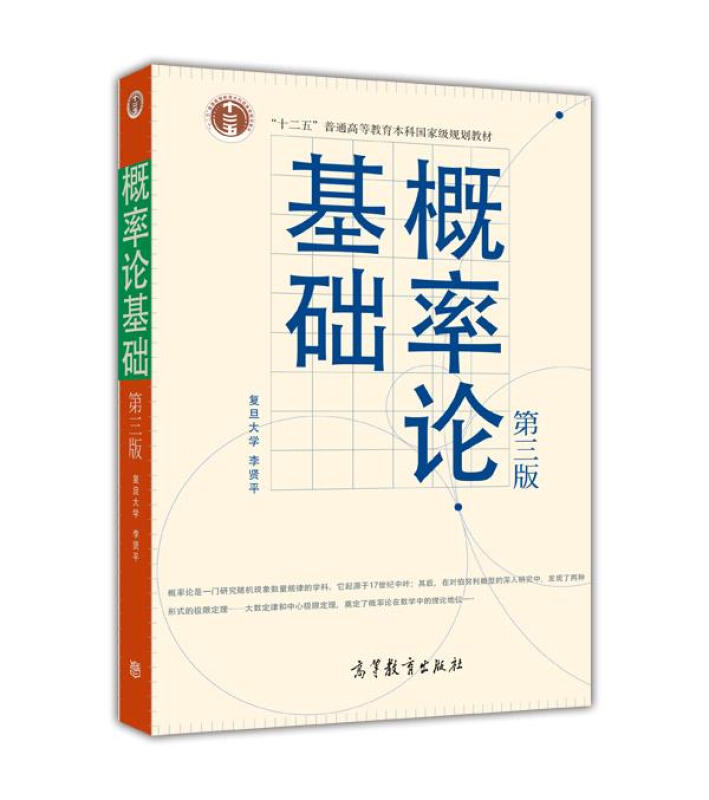Tip

• 《概率论与数理统计》，浙大四版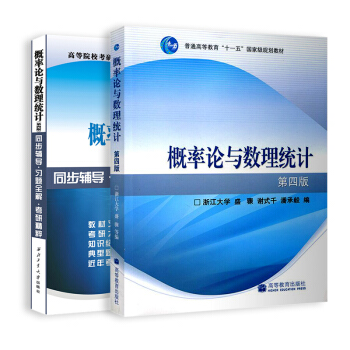Tip

• 《数理统计讲义》，复旦大学，郑明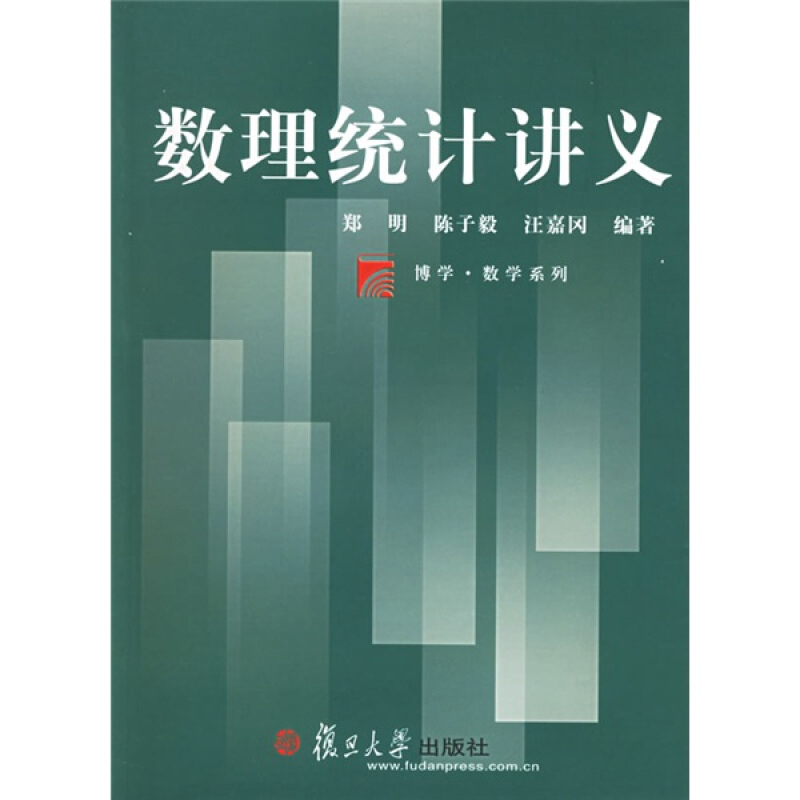Tip

• 《数理统计》，厦门大学，程细玉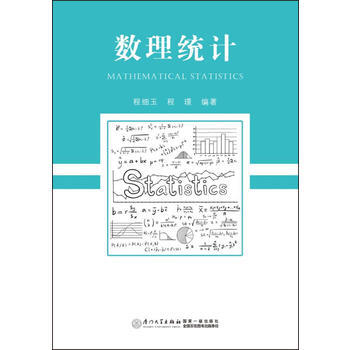Tip

• 《概率论与数理统计教程》，茆诗松，第二版Tip

• Elementary Probability Theory，Kai Lai Chung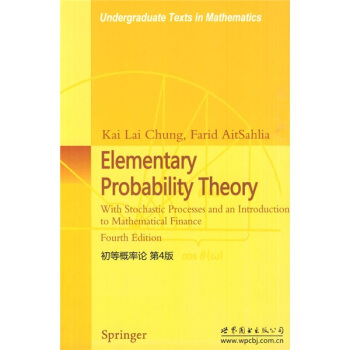Tip

• Introduction to Mathematical Statistics, Hogg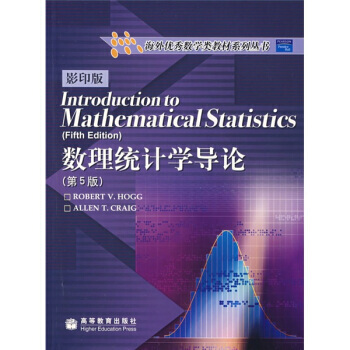Tip

• Statistical Inference, Casella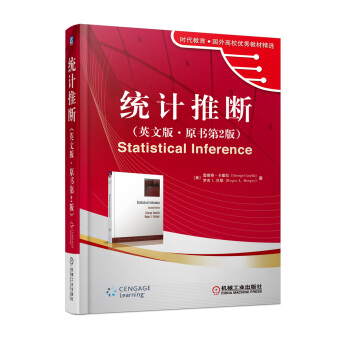Tip

Definition9.1.1 An intervel estimate of a real-valued parameter $$\theta$$ is any pair of functions, $$L(x_{1}, \cdots, x_{n})$$ and $$U(x_{1}, \cdots, x_{n})$$, of a sample that satisfy $$L(\mathbf{x}) \le U(\mathbf{x})$$ for all $$\mathbf{x} \in \mathbf{\chi}$$. If $$\mathbf{\chi} = \mathbf{x}$$ is observed, the inference $$L(\mathbf{x}) \le \theta \le U(\mathbf{x})$$ is made. The random intervel $$[L(\mathbf{X},U(\mathbf{X}]$$ is called an intervel estimate.

• 概率论及数理统计， 中山大学，邓集贤Tip

• An Introduction to Probability and Statistical Inference Second Edition，George G. Roussas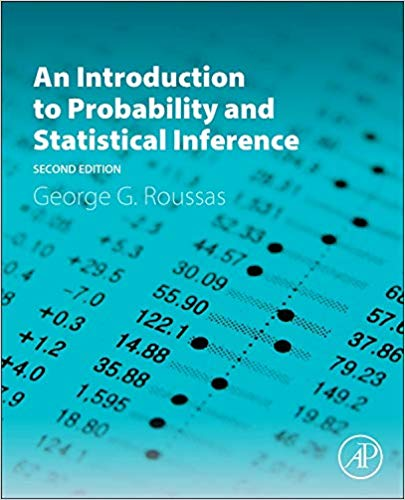Tip